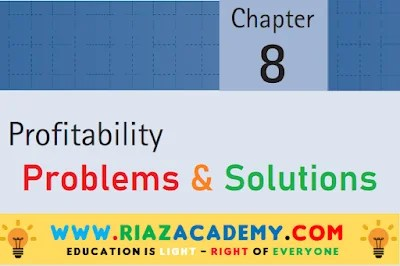# Financial Reporting and Analysis (Chapter # 8 – Profitability) by Charles H. Gibson

## Financial Reporting and Analysis by Charles H. Gibson 13th Edition

IMPORTANT NOTE:

### P  8-1:Ahl Enterprise lists the following data for 2011 and 2010:

Required:
Calculate the net profit margin, return on assets, total asset turnover, and return on common equity for both years. Comment on the results. (For return on assets and total asset turnover, use end-of-year total assets; for return on common equity, use end-of-year common equity.)
Solution:

Ahl Enterprise has had a substantial rise in profit to sales.  This is somewhat tempered by a reduction in asset turnover.  Given a slight rise in common equity, there is a substantial rise in return on common equity.

### P  8-2: Income statement data for Starr Canning Corporation are as follows:

Required:

a.   Prepare an income statement in comparative form, stating each item for both years as a
percent of sales (vertical common-size analysis).
b.   Comment on the findings in (a).
Solution:

B:  Starr Canning has had a sharp decrease in selling expenses coupled with only a modest rise in general expenses giving an overall rise in the net profit margin.

### P  8-3:

The balance sheet for Schultz Bone Company at December 31, 2011 had the following account balances::
Total current liabilities (non-interest-bearing)                  \$450,000
Bonds payable, 6% (issued in 1982; due in 2018)               750,000
Preferred stock, 5%, \$100 par                                              300,000
Common stock, \$10 par                                                         750,000
Retained earnings                                                                   600,000

Income before income tax was \$200,000, and income taxes were \$80,000 for the cur-
rent year.
Required Calculate each of the following:

a. Return on assets (using ending assets)
b. Return on total equity (using ending total equity)
c. Return on common equity (using ending common equity)
d. Times interest earned

Solution:

### P  8-4:  Revenue and expense data for Vent Molded Plastics and for the plastics industry as a whole follow:

Required:
Convert the dollar figures for Vent Molded Plastics into percentages based on net sales. Compare these with the industry average, and comment on your findings.

Solution:

Sales returns are higher than the industry.  Cost of sales is much higher, offset some by lower operating expenses. Other expense (perhaps interest) is somewhat higher.  Lower taxes are perhaps caused by lower income.  Overall profit is less, primarily due to cost of sales.

### P  8-5: Day Ko Incorporated presented the following comparative income statements for 2011 and 2010

Required:

A.  How did 2011 net sales compare with 2010?
B. How did 2011 net earnings compare with 2010?
C. Calculate the following for 2011 and 2010:
1. Net profit margin
2. Return on assets (using ending assets)
3. Total asset turnover (using ending assets)
4. DuPont analysis
5. Operating income margin
6. Return on operating assets (using ending assets)
7. Operating asset turnover (using ending assets)
8. DuPont analysis with operating ratios
9. Return on investment (using ending liabilities and equity)
10. Return on equity (using ending common equity)
D. Based on the previous computations, summarize the trend in profitability for this firm.
Solution:

### P  8-6: Dorex, Inc., presented the following comparative income statements for 2011, 2010, and 2009:

B. All ratios computed indicate a significant improvement in profitability.

P 8-9: The following financial information is for A. Galler Company for 2011, 2010, and 2009:
Required:

a. For 2011, 2010, and 2009, determine the following:
1. Return on assets (using end-of-year total assets)
2. Return on investment (using end-of-year long-term liabilities and equity)
3. Return on total equity (using ending total equity)
4. Return on common equity (using ending common equity)
b. Discuss the trend in these profit figures.
c. Discuss the benefit from the use of long-term debt and preferred stock.

b. Return on assets improved in 2003 and then declined in2004.  Return on investment improved each year.  Return on total equity improved and then declined.  Return on common equity improved and then declined. In general, profitability has improved in 2003 over 2002 but was down slightly in 2004.

c. The use of long-term debt and preferred stock both benefited profitability. Return on common equity is slightly more than return on total equity, indicating a benefit from preferred stock. Return on total equity is substantially higher than return on investment, indicating a benefit from long-term debt.

Might be You Like the Followings:

## ACCA F2 Management Accounting Lecture 86 – Performance Measurement – Introduction

ACCA F2 Management Accounting  Lecture # 86 – Performance Measurement – Introduction Please wait to …